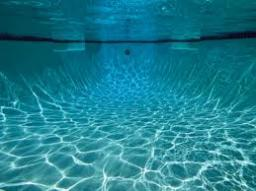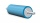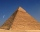# Pool

The swimming pool is 10 m wide and 8 m long and 153 cm deep. How many hectoliters of water is in it, if the water is 30 cm below its upper edge?

Result

V =  984 hl

#### Solution:Our examples were largely sent or created by pupils and students themselves. Therefore, we would be pleased if you could send us any errors you found, spelling mistakes, or rephasing the example. Thank you!

Leave us a comment of this math problem and its solution (i.e. if it is still somewhat unclear...):Math student
IT'S 9 CM NOT 10 CM. SO IT SHOULD BE 4004.Dr Math
corrected, thank youTips to related online calculators
Do you want to convert length units?
Do you know the volume and unit volume, and want to convert volume units?

## Next similar math problems:

1. Swimming poolThe swimming pool has the shape of a block with dimensions of 70dm, 25m, 200cm. How many hl of water can fit into the pool?
2. PoolHow many hl of water is in a cuboid pool (a = 25m, b = 8m) if the area of the wetted walls is 279.2 m2?
3. A filterIt is a pool with a volume of 3500 liters. The filter filters at 4m cubic per hour. How many minutes would it filter the entire pool?
4. A plasticineJožko modeled from plasticine. He used 27g of plasticine to model a 3 cm long cube. How many grams of plasticine will it need to mold cubes with an edge of 6cm?
5. Conical bottleWhen a conical bottle rests on its flat base, the water in the bottle is 8 cm from it vertex. When the same conical bottle is turned upside down, the water level is 2 cm from its base. What is the height of the bottle?
6. CylinderThe 1.8m cylinder contains 2000 liters of water. What area (in dm2) of this container is the water?
7. Hexa pyramidThe base of the regular pyramid is a hexagon, which can be described by a circle with a radius of 1 m. Find the volume of the pyramid 2.5 m high.
8. The ballThe ball has a radius of 2m. What percentage of the surface and volume is another sphere whose radius is 20% larger?The quadrilateral pyramid has a rectangular base of 24 cm x 3.2dm and a body height of 0.4m. Calculate its volume and surface area.
10. The prison ballCalculate the density of the material that the prison ball is made from if you know its diameter is 15cm and its weight is approximately 2.3kg. With the help of mathematical-physicochemical tables estimate what material the ball is made from.
11. Axial section of the coneThe axial section of the cone is an isosceles triangle in which the ratio of cone diameter to cone side is 2: 3. Calculate its volume if you know its area is 314 cm square.
12. Cone sideCalculate the volume and area of the cone whose height is 10 cm and the axial section of the cone has an angle of 30 degrees between height and the cone side.
13. Length of the edgeFind the length of the edge of a cube that has a cm2 surface and a volume in cm3 expressed by the same number.
14. A square baseA solid right pyramid has a square base. The length of the base edge is 4 centimeters and the height of the pyramid is 3 centimeters. What is the volume of the pyramid?
15. Octagonal pyramidFind the volume of a regular octagonal pyramid with height v = 100 and the angle of the side edge with the plane of the base is α = 60°.
16. Tetrahedral pyramidDetermine the surface of a regular tetrahedral pyramid when its volume is V = 120 and the angle of the sidewall with the base plane is α = 42° 30´.
17. Uboid volumeCalculate the cuboid volume if the walls are 30cm², 35cm², 42cm²